# Kerala Plus One Maths Important Questions

Class 11 Mathematics is very important, as it helps students to get a strong foundation for the class 12 maths. Students who have opted for mathematics for their class 11 and 12 are those who aim to do engineering entrance exams and those who wish to take up mathematics for their higher studies. The concepts and topics covered in mathematics as per the Kerala Class 11 syllabus, will be beneficial for students who aim to do competitive exams. These students also plan to prepare ahead for the exams with the help of study resources and Kerala Plus One Maths important questions.

## Why do Students Opt for Kerala Board Class 11 Important Questions in Maths?

Meanwhile, see here some of the advantages of the important questions of Class 11 maths that have been compiled:

• Students get better acquainted with the type of questions asked
• They get more practice and gain more confidence
• They get used to solving questions in mathematics
• It covers important concepts and topics in the subject
• Students are able to study well and prepare for exams

Having recognized the importance of delivering resources study material for the Kerala board plus one students, we at BYJU’s have compiled a list of important questions from Maths subject of the Kerala Board Class 11:

### KBPE Class 11th Maths Important Questions & Answers

Question 1: Find sum to “n” terms of the sequence 4 + 44 + 444 + _________.

Solution:

4 + 44 + 444 + … to n terms

= 4 [ 1 + 11 + 111 + … to n terms ]

= (4 / 9) [ 9 + 99 + 999 + … to n terms ]

= (4 / 9) [(10 – 1) + (10² – 1) + (10³ – 1) + … + (10ⁿ – 1)]

= (4 / 9) [(10 + 10² + 10³ + … + 10ⁿ ) – ( 1 + 1 + 1 + … n times)]

= (4 / 9) {10 [(10ⁿ – 1 ) / ( 10 – 1 ) ] – n(1)}

= (4 / 9) [(10 / 9) ( 10ⁿ – 1 ) – n]

Question 2: Solve sin 2x + sin 4x + sin 6x = 0.

Solution:

(sin 2x + sin 6x) + sin 4x = 0

2 sin 4x . cos2x + sin 4x = 0

sin 4x (2 cos 2x + 1) = 0

sin 4x = 0 or 2 cos 2x + 1= 0

sin 4x = 0 or cos 2x = – 1 / 2 = – cos 𝛑 / 3 = cos (𝛑 – 𝛑 / 3)

Using sinx = 0 ⇒ x = n𝛑

sin 4x = 0

4x = n𝛑

The general solution is x = (n𝛑) / 4 using cos x = cos ɑ

⇒ x = 2mx ± ɑ

cos 2x = cos ((2𝛑) / 3)

2x = 2m𝛑 ± (2𝛑) / 3

The general solution is x = m𝛑 ± 𝛑 / 3 where m,n in z.

Question 3: One card is drawn at random from a pack of 52 playing cards. Find the probability that:

[a] The card drawn is black

[b] The card drawn is a face card

[c] The card drawn is a blackface card

Solution:

[a] There are 26 black cards in a deck.

Let E1 = event of getting a black card

Number of favourable outcomes = 26

P (E1) = Number of favourable outcomes / total number of outcomes

P (E1) = 26 / 52 = 1 / 2

[b] There are 12 face cards.

Let E2 = Event of getting a face card

Number of favourable outcomes to E2 = 12

P(E2) = Number of favourable outcomes / total number of outcomes

P(E2) = 12 / 52 = 3 / 13

[c] The probability of choosing a black face card is 6 / 52.

Question 4: (a) If A= {a, b, c}, then write the power set P (A).

(b) If the number of subsets with 2 elements of a set P is 10, then find the total number of elements in set P.

(c) Find the number of elements in the power set of P.

Solution:

[a] P(A) = {○ , {a} , {b} , {c} , {a , b} , {b , c} , {c , a} , {a , b , c} }

[b] Number of subsets with 2 elements = nC2

nC2 = 10

n (n – 1) ÷ 2 = 10

n (n – 1) = 20

n (n – 1) = 5 (5 – 1)

n = 5

[c] 2n

Question 5: Consider the Venn diagram of the Universal Set U = {1, 2, 3, 4, 5, 6, 7, 8, 9, 10, 11, 12, 13}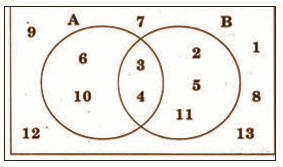[a] Write sets A and B in Roster form

[b] Verify (A ∪ B)′ = A′ ∩ B′

Solution:

[a] A = {6, 10, 3, 4}

B = {2, 3, 4, 5, 11}

[b] A ∪ B = {2, 3, 4, 5, 6, 10, 11}

(A ∪ B)’ = {1, 7, 8, 9, 12, 13}

A’ = {1, 2, 5, 7, 8, 9, 12, 13}

B’ = {1, 6, 7, 8, 9, 10, 12, 13}

A′ ∩ B′ = {1, 7, 8, 9, 12, 13}

(A ∪ B)′ = A′ ∩ B′

Question 6: The figure shows the graph of a function f(x), which is a semi-circle centred at the origin: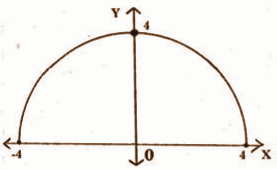[a] Write the domain and range of the function.

[b] Define the function f (x).

Solution:

[a] Domain = [- 4, 4]

Range = [0, 4] [b] x2 + y2 = 16

y2 = 16 – x2

y = √16 – x2

f (x) = √16 – x2

Question 7: Consider a point A (4, 8, 10 ) in space.

[a] Find the distance of the point A from XY – plane.

[b] Find the distance of point A from the x-axis.

[c] Find the ratio in which the line segment joining the point A and B (6, 10, -8) is divided by YZ- plane.

Solution:

[a] 10

[b] Let P (4, 0, 0) be a line on the x-axis.

Distance = √(4 – 4)2 + (8 – 0)2 + (10 – 0)2 = 2√41

[c] [mx2 + nx1] / [m + n] [mx2 + nx1] = 0

m / n = – x1 / x2 = – 2 / 3

m:n = – 2:3

Question 8: Consider the quadratic equation, x2 + x + 1 = 0

[b] Write the polar form of one of the roots

[c] If the two roots of the given quadratic are ɑ and β, show that ɑ2 = β.

Solution:

[a] x2 + x + 1 = 0

x = – b ± √b2 – 4ac / 2a

x = – 1 ± √3i / 2

[b] Let Z = (- 1 / 2) + i √3 / 2

x = – 1 / 2 and y = √3 / 2

r = √x2 + y2 = √(1 / 4) + (3 / 4) = 1

θ = 𝛑 – (𝛑 / 3) = 2𝛑 / 3

Z = r (cos θ + i sin θ)

= 1 (cos (2𝛑 / 3) + i sin (2𝛑 / 3)) is the polar form

Question 9: Consider the following data: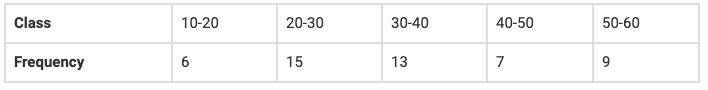[a] Calculate the mean of the distribution.

[b] Find the standard deviation of the distribution.

[c] Find the coefficient of variation of the distribution.

Solution:

[a]
 Class fi xi fixi xi2 fixi2 10 – 20 6 15 90 225 1350 20 – 30 15 25 375 625 9375 30 – 40 13 35 455 1225 15925 40 – 50 7 45 315 2025 14175 50 – 60 9 55 495 3025 27225 50 1730 68050
[a] Mean = ∑fixi / ∑fi

= 1730 / 50

= 34.6

[b] Variance = ∑fixi2 / ∑fi – (mean)2

= [68050 / 50] – [34.6]2

= 163.84

Standard deviation = 12.8

[c] Coefficient of variation = [SD / (mean)] * 100

= (12.8 / 34.6) * 100

= 36.99

Question 10: Consider the statement “102n−1 + 1 is divisible by 11”. Verify that P(1) is true and then prove the statement by using mathematical induction.

Solution:

P (1) = 102n−1 + 1 = 102-1 + 1 = 10 + 1 = 11 is divisible by 11.

Hence P (1) is true.

P (k) = 102k-1 + 1 is divisible by 11

102k-1 + 1 = 11d

102k-1 = 11d – 1 —- (1)

To prove that P (k + 1) is true

P (k + 1) = 102(k+1)-1 + 1

102k+2-1 + 1 = 102k-1+2 + 1

= 102k-1 . 102 + 1

= 100 . 102k-1 + 1

= 100 [11d – 1] + 1

= 100 [11d] – 100 + 1

= 100 [11d] – 99

= 11 [100d – 9]

= 11d

P (k + 1) is true.

Hence P(n) is true for all n ∈ N.

Question 11[a]: Solve the inequality x / 3 > x / 2 + 1

(b) Solve the system of inequalities graphically:

2x + y > 6

3x + 4y ≤ 12

Solution:

[a] x / 3 > x / 2 + 1

On multiplying by 6,

6 (x / 3) > 6 (x / 2) + 6 (1)

2x > 3x + 6

2x – 3x > 6

– x > 6

x < -6

x = (- ∞, – 6)

[b]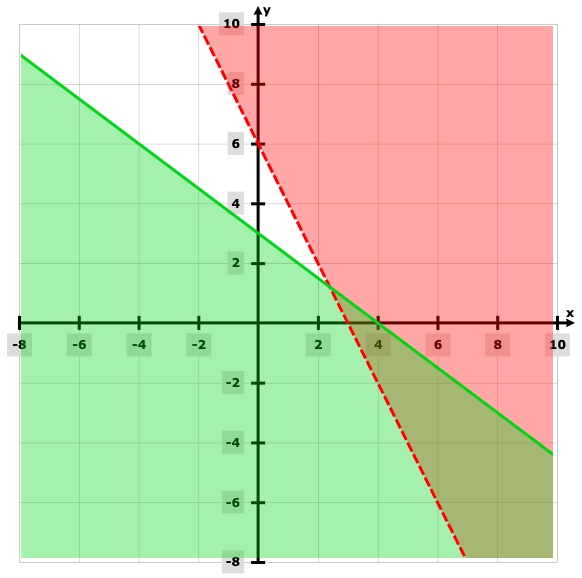Question 12[a]: The distance between the points (1, -2, 3) and (4, 1, 2) is ______

[i] √12

[ii] √19

[iii] √11

[iv] √15

[b] The centroid of a triangle ABC is at the point (1, 2, 3). If the coordinates of A and B are (3, -5, 7) and (-1, 7, -6) respectively. Find the coordinate points of C.

Solution: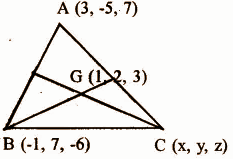[3 + (-1) + x] / 3

⇒ 2 + x = 3

⇒ x = 3 – 2 = 1

[-5 + 7 + y] / 3

⇒ 2 + y = 6

⇒ y = 6 – 2 = 4

[7 + (-6) + z] / 3

⇒ 1 + z = 9

⇒ z = 9 – 1 = 8

The coordinates of C are (1, 4, 8).

Question 13: [a] Find the variance for the observations 2,4,6,8 and 10.

(b) Consider the frequency distribution

 x 5 10 15 20 25 f 7 4 6 3 5

[i] Find the mean.

[ii] Find the mean deviation about the mean.

Solution:

[a]
 x 5 10 15 20 25 f 7 4 6 3 5 fx 35 40 90 60 125 x – (mean) 9 4 1 6 11 f [x – (mean)] 63 16 6 18 55
[i] Mean = ∑fixi / ∑fi

= 350 / 25

= 14

[ii] Mean deviation about mean = ∑fi |x – (mean)| / N

= 158 / 25

= 6.32

Question 14[i]: sin 225°_________

(a) 1 / √2

(b) √3 / 2

(c) − 1 / √2

(d) 1 / 2

(ii) Find the principal and general solutions of sin x = – √3 / 2

(iii) Prove that tan [A − B / 2] = [a − b] / [a + b] cot c / 2.

Solution:

[ii] sin x = – √3 / 2

= – sin (𝛑 / 3)

= sin (𝛑 + 𝛑 / 3)

= sin (4𝛑 / 3)

sin x = sin (4𝛑 / 3)

x = n𝛑 + (- 1)n (4𝛑 / 3)

[c] From the law of cosines a = R sin A, b = R sin B

(a – b) / (a + b) = [2R sin A – 2R sin B] / [2R sin A + 2R sin B]

= [sin A – sin B] / [sin A + sin B]

= [2 cos (A + B) / 2 * sin (A – B) / 2] / [2 sin (A + B) / 2 * cos (A – B) / 2]

= cot (A + B) / 2 * tan (A – B) / 2

= cot (90 – C / 2) * tan (A – B) / 2

= tan C / 2 * tan (A – B) / 2

= 1 / cot (C / 2) * tan (A – B) / 2

tan (A – B) / 2 = [(a – b) / (a + b)] cot C / 2

Question 15[a]: Find the equation of the line passing through the points (3, -2) and (-1, 4).

(b) Reduce the equation √3x + y − 8 = 0 into normal form.

(c) If the angle between two lines is π / 4 and the slope of one of the lines is 1 / 2, find the slope of the other line.

Solution:

[a] y – y1 = [y2 – y1 / x2 – x1] (x – x1)

y + 2 = [4 + 2] / [- 1 – 3] [x – 3]

y + 2 = (- 3 / 2) (x – 3)

2y + 4 = – 3x + 9

3x + 2y – 5 = 0

[b] √3x + y − 8 = 0

√3x + y = 8

Dividing by (√3)2 + 12 = 2

(√3 / 2) x + (1 / 2) y = 4

[c] cos 30o x + sin 30o y = 4

m1 = (1 / 2)

m2 = m1

tan θ = |(m2 – m1) / (1 + m1m2)|

tan 𝛑 / 4 = |(m2 – (1 / 2)) / 1 + (1 / 2) m2|

m = 3 or (- 1 / 3)

Question 16: [a] Which one among the following is the interval corresponding to the inequality: −2 < x ≤ 3?

(a) [-2, 3]

(b) [ -2, 3)

(c) (-2, 3]

(d) (-2, 3)

(b) Solve the following inequalities graphically:

2x + y ≥ 4

x + y ≤ 3

2x − 3y ≤ 6

Solution:

[a] (-2, 3] [b]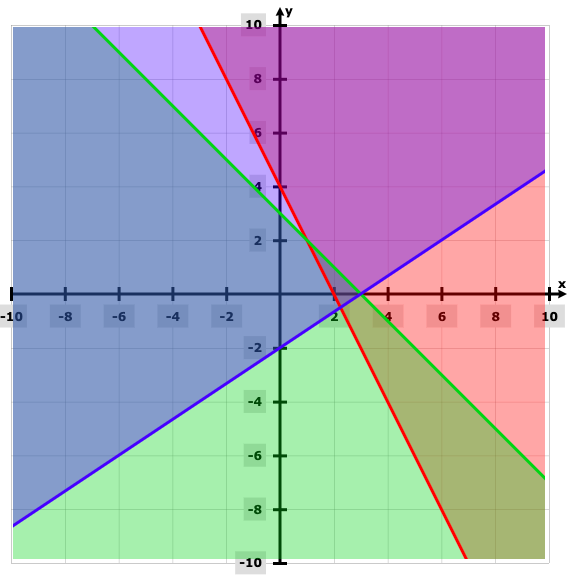Question 17: (a) Write the negation of the statement :

“Every natural number is greater than zero”

(b) Verify by the method of contradiction :

“P: √13 is irrational”.

Solution:

[a] Negation: It is false that every natural number is greater than 0. There exists a natural number which is not greater than 0.

[b] Assume that √13 is rational, √13 = (a / b), where a and b are coprime.

13b2 = a2

13 divides a

There exists an integer k such that a = 13k.

a2 = 169k2

13b2 = 169k2

13k2 = 13 divides b

13 divides both a and b which is a contradiction to our assumption that √13 is rational. So, √13 is irrational.

Question 18: (a) A= { X / X is a prime number, X ≤ 6

(i) Represent A in the Roster form

(ii) Write the Powerset of A

(b) Out of the 25 members in an office, 17 like to take tea, 16 like to take coffee. Assume that each takes at least one of the two drinks.

How many like:

(i) Both Coffee and Tea?

(ii) Only Tea and not Coffee?

Solution:

[a] [i] Roster form = {2,3,5}

[ii] Power set = {( ), (2), (3), (5), (2, 3), (2, 5) (5, 3), (2, 3, 5)}

[b] [i] Let –

A = {people drinking tea}

B = {people drinking coffee}

A ∩ B = {people drinking tea / coffee}

A ∪ B = {people drinking both tea / coffee}

n (A) = 17

n (B) = 16

n (A ∪ B) = 25

Number of people drinking both tea & coffee,

n (A ∩ B) = n (A) + n (B) – n (A ∪ B)

n (A ∩ B) = 17 + 16 – 25

n (A ∩ B) = 8

[ii] Number of people drinking only tea,

n (A / B) = n (A) – n (A ∩ B)

n (A / B) = 17 – 8

n (A / B) = 9

Therefore, the number of people drinking both tea & coffee is 8 and those drinking only tea are 9.

Question 19: (a) Number of terms in the expansion of [x + 1 / x]10 is ______

(i) 10

(ii) 9

(iii) 11

(iv) 12

(b) Find the term independent of x in the above expansion.

Solution:

[b] a = x, b = (1 / x), n = 10

tr+1 = nCr an+r br = 10Cr x10+r (1 / x)r

= 10Cr x10-r (x)-r

= 10Cr x10-r-r

= 10Cr x10-2r

Put 10 – 2r = 0

10 = 2r

r = 5

t5+1 = 10C5 x10-2*5

t6 = 252

Question 20: (i) If the nth term of the sequence is n (n2 + 5) / 4, then find its first two terms.

[ii] How many terms of an AP -6, (11 / 2), -5, …… are needed to give the sum of -25.

[iii] Find the 10th term of a GP whose 3rd term is 24 and 6th term is 192.

Solution:

[i] a1 = 3 / 2; a2 = 9 / 2

[ii] Sn = (n / 2) (2a + [n – 1] d)

-25 = (n / 2) [2 * (-6) + [n – 1] (1 / 2)]

n = 20, 5

[iii] ar2 = 24, ar5 = 192

a = 6, r = 2

a10 = ar9

6 (2)9 = 3072

Question 21: Find the coordinates of the foci vertices, eccentricity and the length of the latus rectum of the ellipse 100x2 + 25y2 = 2500.

Solution:

x2 / 25 + y2 / 100 = 1

The major axis is vertical.

a = 10; b = 5

c2 = a2 – b2 = 75

The foci are at (0, ± √75).